# RD Sharma Solutions for Class 10 Maths Chapter 6 Trigonometric Identities

## RD Sharma Solutions Class 10 Maths Chapter 6 – Free PDF Download

RD Sharma Solutions for Class 10 Maths Chapter 6 – Trigonometric Identities are provided here. The branch of Mathematics which deals with the measurement of the sides and the angles of a triangle is known as trigonometry. Students who find it difficult to understand the concepts covered in this chapter can make their learning process smooth and easy using RD Sharma Solutions. These solutions are well structured by our subject expert team at BYJU’S to help students grasp the in-depth knowledge of concepts which are vital for examinations.

Trigonometric Identities is the 6th Chapter of RD Sharma Solutions Class 10. This chapter consists of two exercises. Students can find the precise answers for these exercises in RD Sharma Solutions for Class 10. The previous chapter was about trigonometric ratios and the relations between them. But this chapter explains the trigonometric identities in a comprehensive manner in accordance with the student’s intelligence quotient.

## RD Sharma Solutions for Class 10 Maths Chapter 6 Trigonometric Identities Here

### RD Sharma Solutions for Class 10 Maths Chapter 6 Exercise 6.1 Page No: 6.43

Prove the following trigonometric identities:

1. (1 – cos2 A) cosec2 A = 1

Solution:

Taking the L.H.S.,

(1 – cos2 A) cosec2 A

= (sin2 A) cosec2 A [∵ sin2 A + cos2 A = 1 ⇒1 – sin2 A = cos2 A]

= 12

= 1 = R.H.S.

– Hence, proved.

2. (1 + cot2 A) sin2 A = 1

Solution:

By using the identity,

cosec2 A – cot2 A = 1 ⇒ cosec2 A = cot2 A + 1

Taking,

L.H.S. = (1 + cot2 A) sin2 A

= cosec2 A sin2 A

= (cosec A sin A)2

= ((1/sin A) × sin A)2

= (1)2

= 1

= R.H.S.

– Hence, proved.

3. tan2 θ cos2 θ = 1 − cos2 θ

Solution:

We know that,

sinθ + cosθ = 1

Taking,

L.H.S. = tanθ cosθ

= (tan θ × cos θ)2

= (sin θ)2

= sin2 θ

= 1 – cos2 θ

= R.H.S.

– Hence, proved.

4. cosec θ √(1 – cos2 θ) = 1

Solution:

Using identity,

sinθ + cosθ = 1  ⇒ sinθ = 1 – cosθ

Taking L.H.S.,

L.H.S = cosec θ √(1 – cos2 θ)

= cosec θ √( sinθ)

= cosec θ x sin θ

= 1

= R.H.S.

– Hence, proved.

5. (sec2 θ − 1)(cosec2 θ − 1) = 1

Solution:

Using identities,

(sec2 θ − tan2 θ) = 1 and (cosec2 θ − cot2 θ) = 1

We have,

L.H.S. = (sec2 θ – 1)(cosec2θ – 1)

= tan2θ × cot2θ

= (tan θ × cot θ)2

= (tan θ × 1/tan θ)2

= 12

= 1

= R.H.S.

– Hence, proved.

6. tan θ + 1/ tan θ = sec θ cosec θ

Solution:

We have,

L.H.S. = tan θ + 1/ tan θ

= (tan2 θ + 1)/ tan θ

= sec2 θ / tan θ [∵ sec2 θ − tan2 θ = 1]

= (1/cos2 θ) x 1/ (sin θ/cos θ) [∵ tan θ = sin θ / cos θ]

= cos θ/ (sin θ x cos2 θ)

= 1/ cos θ x 1/ sin θ

= sec θ x cosec θ

= sec θ cosec θ

= R.H.S.

– Hence, proved.

7. cos θ/ (1 – sin θ) = (1 + sin θ)/ cos θ

Solution:

We know that,

sin2 θ + cos2 θ = 1

So, by multiplying both the numerator and the denominator by (1+ sin θ), we get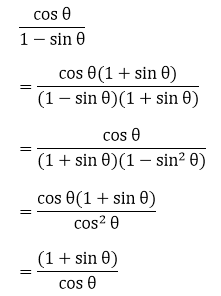L.H.S. = R.H.S.

– Hence, proved.

8. cos θ/ (1 + sin θ) = (1 – sin θ)/ cos θ

Solution:

We know that,

sin2 θ + cos2 θ = 1

So, by multiplying both the numerator and the denominator by (1- sin θ), we get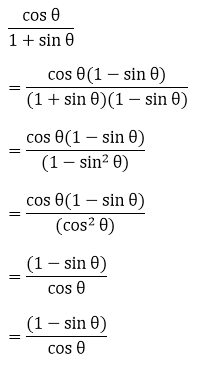L.H.S. = R.H.S.

– Hence, proved.

9. cos2 θ + 1/(1 + cot2 θ) = 1

Solution:

cosec2 θ − cot2 θ = 1 and sin2 θ + cos2 θ = 1

Taking L.H.S.,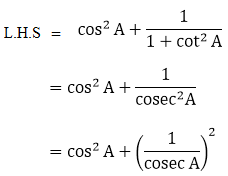= cos2 A + sin2 A

= 1

= R.H.S.

– Hence, proved.

10. sin2 A + 1/(1 + tan 2 A) = 1

Solution:

sec2 θ − tan2 θ = 1 and sin2 θ + cos2 θ = 1

Taking L.H.S.,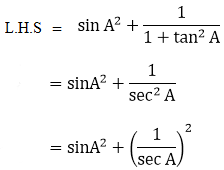= sin2 A + cos2 A

= 1

= R.H.S.

– Hence, proved.

11.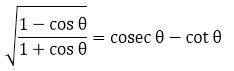Solution:

We know that,

sin2 θ + cos2 θ = 1

Taking the L.H.S.,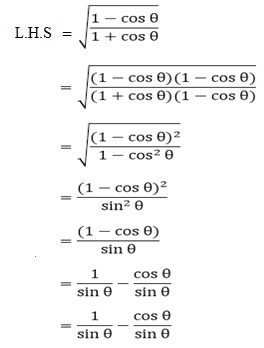= cosec θ – cot θ

= R.H.S.

– Hence, proved.

12. 1 – cos θ/ sin θ = sin θ/ 1 + cos θ

Solution:

We know that,

sin2 θ + cos2 θ = 1

So, by multiplying both the numerator and the denominator by (1+ cos θ), we get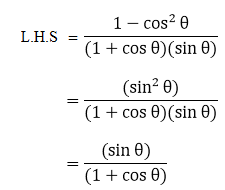= R.H.S.

– Hence, proved.

13. sin θ/ (1 – cos θ) = cosec θ + cot θ

Solution:

Taking L.H.S.,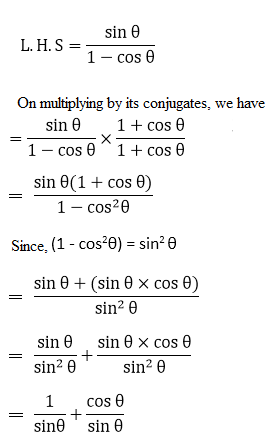= cosec θ + cot θ

= R.H.S.

– Hence, proved.

14. (1 – sin θ) / (1 + sin θ) = (sec θ – tan θ)2

Solution:

Taking the L.H.S.,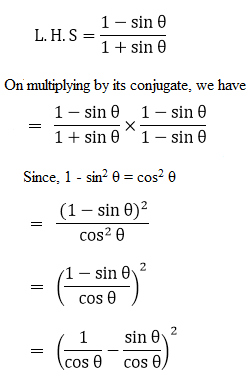= (sec θ – tan θ)2

= R.H.S.

– Hence, proved.

15.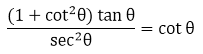Solution:

Taking L.H.S.,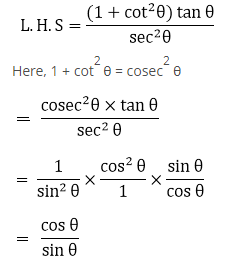= cot θ

= R.H.S.

– Hence, proved.

16. tan2 θ − sin2 θ = tan2 θ sin2 θ

Solution:

Taking L.H.S.,

L.H.S = tan2 θ − sin2 θ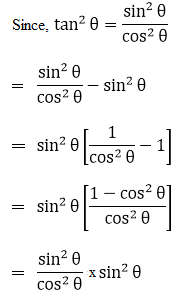= tan2 θ sin2 θ

= R.H.S.

– Hence, proved.

17. (cosec θ + sin θ)(cosec θ – sin θ) = cot2θ + cos2θ

Solution:

Taking L.H.S. = (cosec θ + sin θ)(cosec θ – sin θ)

On multiplying, we get

= cosec2 θ – sin2 θ

= (1 + cot2 θ) – (1 – cos2 θ) [Using cosec2 θ − cot2 θ = 1 and sin2 θ + cos2 θ = 1]

= 1 + cot2 θ – 1 + cos2 θ

= cot2 θ + cos2 θ

= R.H.S.

– Hence, proved.

18. (sec θ + cos θ) (sec θ – cos θ) = tan2 θ + sin2 θ

Solution:

Taking L.H.S. = (sec θ + cos θ)(sec θ – cos θ)

On multiplying, we get,

= sec2 θ – sin2 θ

= (1 + tan2 θ) – (1 – sin2 θ) [Using sec2 θ − tan2 θ = 1 and sin2 θ + cos2 θ = 1]

= 1 + tan2 θ – 1 + sin2 θ

= tan 2 θ + sin 2 θ

= R.H.S.

– Hence, proved.

19. sec A(1- sin A) (sec A + tan A) = 1

Solution:

Taking L.H.S. = sec A(1 – sin A)(sec A + tan A)

Substituting sec A = 1/cos A and tan A =sin A/cos A in the above, we have,

L.H.S = 1/cos A (1 – sin A)(1/cos A + sin A/cos A)

= 1 – sin2 A / cos2 A [After taking L.C.M]

= cos2 A / cos2 A [∵ 1 – sin2 A = cos2 A]

= 1

= R.H.S.

– Hence, proved.

20. (cosec A – sin A)(sec A – cos A)(tan A + cot A) = 1

Solution:

Taking L.H.S. = (cosec A – sin A)(sec A – cos A)(tan A + cot A)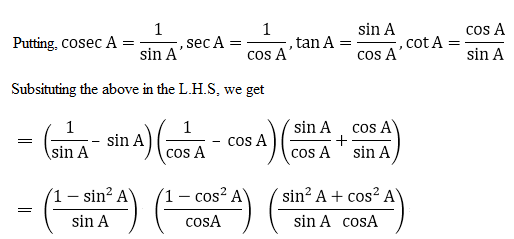= (cos2 A/ sin A) (sin2 A/ cos A) (1/ sin A cos A) [∵ sin2 θ + cos2 θ = 1]

= (sin A  cos A)  (1/ cos A sin A)

= 1

= R.H.S.

– Hence, proved.

21. (1 + tan2 θ)(1 – sin θ)(1 + sin θ) = 1

Solution:

Taking L.H.S. = (1 + tan2θ)(1 – sin θ)(1 + sin θ)

And, we know sin2 θ + cos2 θ = 1 and sec2 θ – tan2 θ = 1

So,

L.H.S = (1 + tan2 θ)(1 – sin θ)(1 + sin θ)

= (1 + tan2 θ){(1 – sin θ)(1 + sin θ)}

= (1 + tan2 θ)(1 – sin2 θ)

= sec2 θ (cos2 θ)

= (1/ cos2 θ) x cos2 θ

= 1

= R.H.S.

– Hence, proved.

22. sin2 A cot2 A + cos2 A tan2 A = 1

Solution:

We know that,

cot2 A = cos2 A/ sin2 A and tan2 A = sin2 A/cos2 A

Substituting the above in L.H.S., we get

L.H.S = sin2 A cot2 A + cos2 A tan2 A

= {sin2 A (cos2 A/ sin2 A)} + {cos2 A (sin2 A/cos2 A)}

= cos2 A + sin2 A

= 1 [∵ sin2 θ + cos2 θ = 1]

= R.H.S.

– Hence, proved.

23.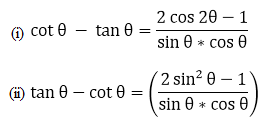Solution:

(i) Taking the L.H.S and using sin2 θ + cos2 θ = 1, we have

L.H.S = cot θ – tan θ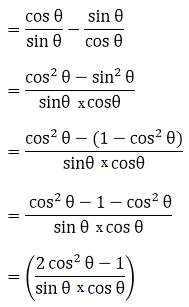= R.H.S.

– Hence, proved.

(ii) Taking the L.H.S. and using sin2 θ + cos2 θ = 1, we have

L.H.S. = tan θ – cot θ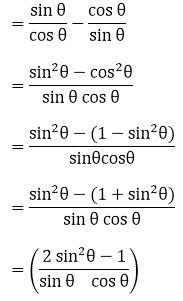= R.H.S.

– Hence, proved.

24. (cos2 θ/ sin θ) – cosec θ + sin θ = 0

Solution:

Taking L.H.S. and using sin2 θ + cos2 θ = 1, we have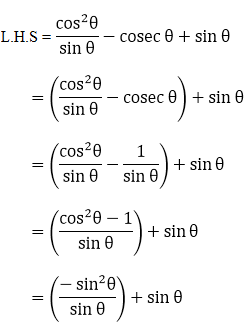= – sin θ + sin θ

= 0

= R.H.S.

– Hence, proved.

25.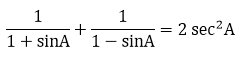Solution:

Taking L.H.S.,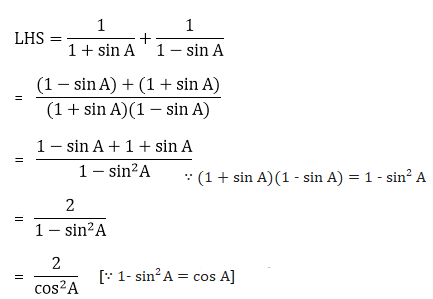= 2 sec2 A

= R.H.S.

– Hence, proved.

26.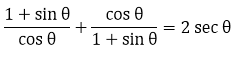Solution:

Taking the LHS and using sin2 θ + cos2 θ = 1, we have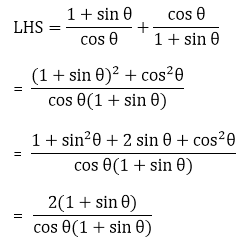= 2/ cos θ

= 2 sec θ

= R.H.S.

– Hence, proved.

27.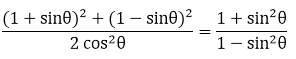Solution:

Taking the LHS and using sin2 θ + cos2 θ = 1, we have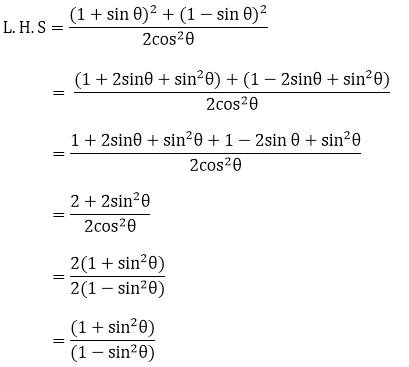= R.H.S.

• Hence, proved.

28.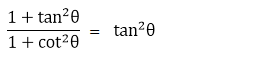Solution:

Taking L.H.S.,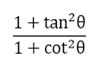Using sec2 θ − tan2 θ = 1 and cosec2 θ − cot2 θ = 1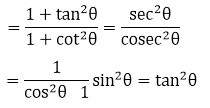= R.H.S.

– Hence, proved.

29.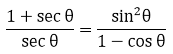Solution:

Taking L.H.S. and using sin2 θ + cos2 θ = 1, we have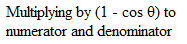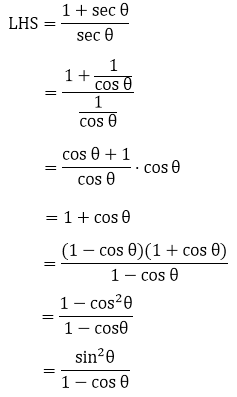= R.H.S.

– Hence, proved.

30.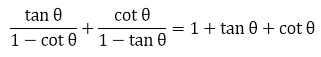Solution:

Taking LHS, we have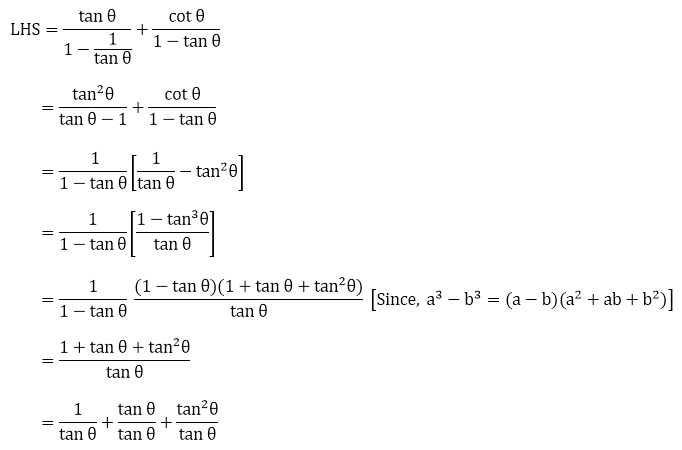= 1 + tan θ + cot θ

= R.H.S.

– Hence, proved.

31. sec6 θ = tan6 θ + 3 tanθ sec2 θ + 1

Solution:

From trig. identities we have,

sec2 θ − tan2 θ = 1

On cubing both sides,

(sec2θ − tan2θ)3 = 1

sec6 θ − tan6 θ − 3sec2 θ tan2 θ(sec2 θ − tan2 θ) = 1

[Since, (a – b)3 = a3 – b3 – 3ab(a – b)]

sec6 θ − tan6 θ − 3sec2 θ tan2 θ = 1

⇒ sec6 θ = tan6 θ + 3sec2 θ tan2 θ + 1

L.H.S. = R.H.S.

Hence, proved.

32. cosec6 θ = cot6 θ + 3cot2 θ cosec2 θ + 1

Solution:

From trig. identities we have,

cosec2 θ − cot2 θ = 1

On cubing both sides,

(cosec2 θ − cot2 θ)3 = 1

cosec6 θ − cot6 θ − 3cosec2 θ cot2 θ (cosec2 θ − cot2 θ) = 1

[Since, (a – b)3 = a3 – b3 – 3ab(a – b)]

cosec6 θ − cot6 θ − 3cosec2 θ cot2 θ = 1

⇒ cosec6 θ = cot6 θ + 3 cosec2 θ cot2 θ + 1

L.H.S. = R.H.S.

Hence, proved.

33.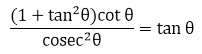Solution:

Taking L.H.S. and using sec2 θ − tan2 θ = 1 ⇒ 1 + tan2 θ = sec2 θ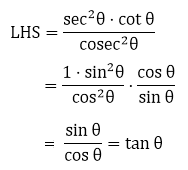= R.H.S.

– Hence, proved.

34.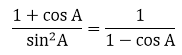Solution:

Taking L.H.S. and using the identity sin2A + cos2A = 1, we get

sin2A = 1 − cos2A

⇒ sin2A = (1 – cos A)(1 + cos A)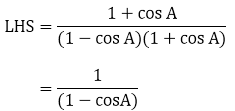Hence, proved.

35.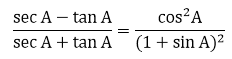Solution:

We have,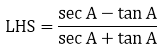Rationalising the denominator and numerator with (sec A + tan A) and using sec2 θ − tan2 θ = 1, we get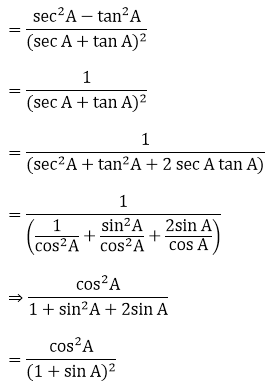= R.H.S.

– Hence, proved.

36.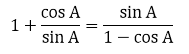Solution:

We have,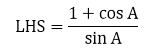On multiplying the numerator and denominator by (1 – cos A), we get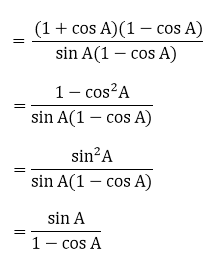= R.H.S.

– Hence, proved.

37. (i)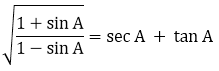Solution:

Taking L.H.S. and rationalising the numerator and denominator with √(1 + sin A), we get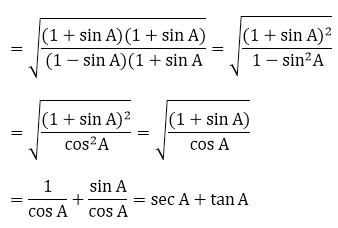= R.H.S.

Hence, proved.

(ii)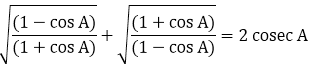Solution:

Taking L.H.S. and rationalising the numerator and denominator with their respective conjugates, we get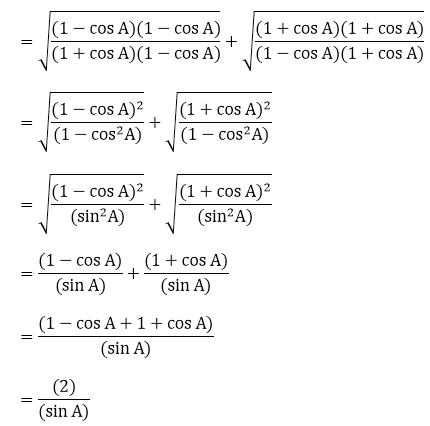= 2 cosec A

= R.H.S.

– Hence, proved.

38. Prove that:

(i)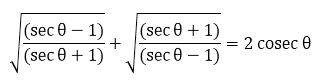Solution:

Taking L.H.S. and rationalising the numerator and denominator with their respective conjugates, we get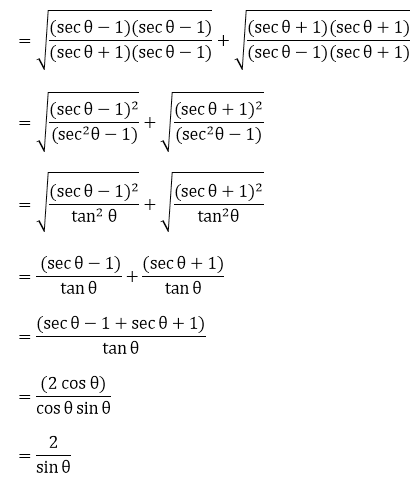= 2 cosec θ

= R.H.S.

– Hence, proved.

(ii)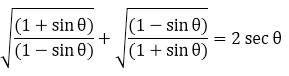Solution:

Taking L.H.S. and rationalising the numerator and denominator with their respective conjugates, we get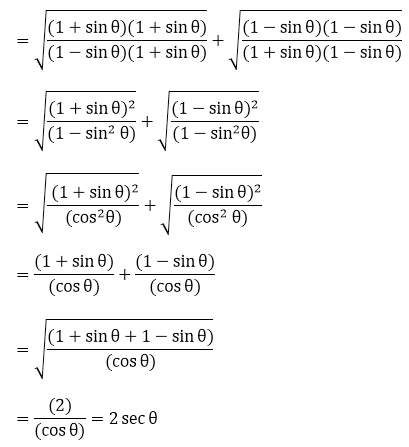= R.H.S.

– Hence, proved.

(iii)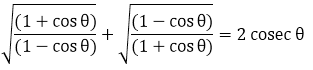Solution:

Taking L.H.S. and rationalising the numerator and denominator with their respective conjugates, we get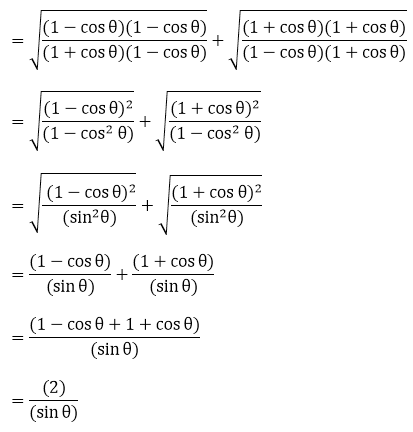= 2 cosec θ

= R.H.S.

– Hence, proved.

(iv)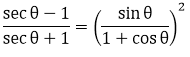Solution:

Taking L.H.S., we have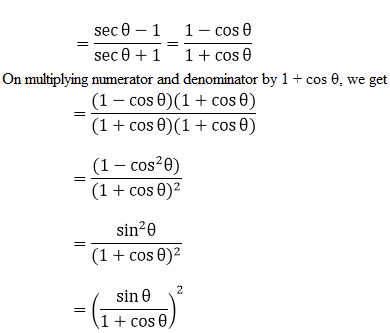= R.H.S.

– Hence, proved.

39.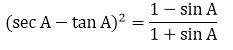Solution:

Taking LHS = (sec A – tan A)2, we have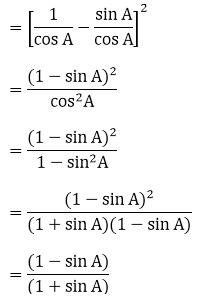= R.H.S.

– Hence, proved.

40.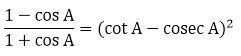Solution:

Taking L.H.S. and rationalising the numerator and denominator with (1 – cos A), we get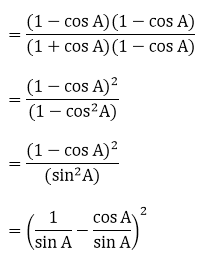= (cosec A – cot A)2

= (cot A – cosec A)2

= R.H.S.

– Hence, proved.

41.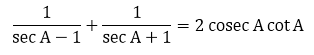Solution:

Considering L.H.S. and taking L.C.M. and on simplifying, we have,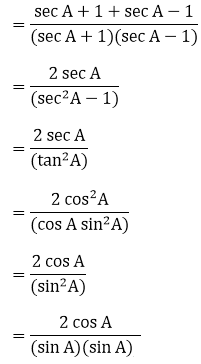= 2 cosec A cot A = RHS

Hence, proved.

42.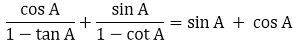Solution:

Taking LHS, we have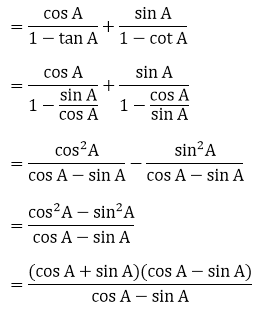= cos A + sin A

= RHS

– Hence, proved.

43.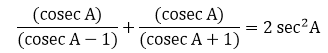Solution:

Considering L.H.S. and taking L.C.M. and on simplifying, we have,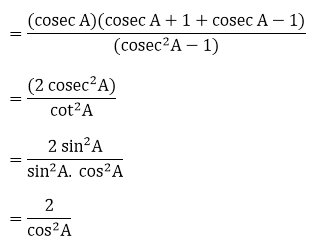= 2 sec2 A

= RHS

Hence, proved.

### RD Sharma Solutions for Class 10 Maths Chapter 6 Exercise 6.2 Page No: 6.54

1. If cos θ = 4/5, find all other trigonometric ratios of angle θ.

Solution:

We have,

cos θ = 4/5

And we know that,

sin θ = √(1 – cos2 θ)

⇒ sin θ = √(1 – (4/5)2)

= √(1 – (16/25))

= √[(25 – 16)/25]

= √(9/25)

= 3/5

∴ sin θ = 3/5

Since, cosec θ = 1/ sin θ

= 1/ (3/5)

⇒ cosec θ = 5/3

And, sec θ = 1/ cos θ

= 1/ (4/5)

⇒ cosec θ = 5/4

Now,

tan θ = sin θ/ cos θ

= (3/5)/ (4/5)

⇒ tan θ = 3/4

And, cot θ = 1/ tan θ

= 1/ (3/4)

⇒ cot θ = 4/3

2. If sin θ = 1/√2, find all other trigonometric ratios of angle θ.

Solution:

We have,

sin θ = 1/√2

And we know that,

cos θ = √(1 – sin2 θ)

⇒ cos θ = √(1 – (1/√2)2)

= √(1 – (1/2))

= √[(2 – 1)/2]

= √(1/2)

= 1/√2

∴ cos θ = 1/√2

Since, cosec θ = 1/ sin θ

= 1/ (1/√2)

⇒ cosec θ = √2

And, sec θ = 1/ cos θ

= 1/ (1/√2)

⇒ sec θ = √2

Now,

tan θ = sin θ/ cos θ

= (1/√2)/ (1/√2)

⇒ tan θ = 1

And, cot θ = 1/ tan θ

= 1/ (1)

⇒ cot θ = 1

3.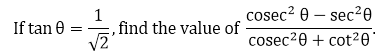Solution:

Given,

tan θ = 1/√2

By using sec2 θ − tan2 θ = 1,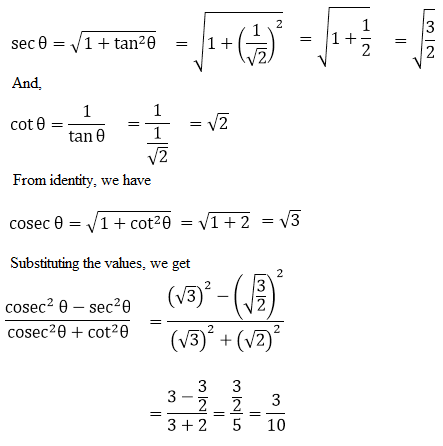4.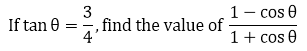Solution:

Given,

tan θ = 3/4

By using sec2 θ − tan2 θ = 1,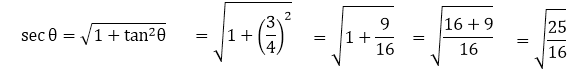sec θ = 5/4

Since, sec θ = 1/ cos θ

⇒ cos θ = 1/ sec θ

= 1/ (5/4)

= 4/5

So,

$$\begin{array}{l}\frac{1-cos\theta }{1+cos\theta }=\frac{1-\frac{4}{5}}{1+\frac{4}{5}}=\frac{\frac{1}{5}}{\frac{9}{5}}=\frac{1}{9}\end{array}$$

5.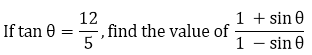Solution:

Given, tan θ = 12/5

Since, cot θ = 1/ tan θ = 1/ (12/5) = 5/12

Now, by using cosec2 θ − cot2 θ = 1,

cosec θ = √(1 + cot2 θ)

= √(1 + (5/12)2 )

= √(1 + 25/144)

= √(169/ 25)

⇒ cosec θ = 13/5

Now, we know that

sin θ = 1/ cosec θ

= 1/ (13/5)

⇒ sin θ = 5/13

Putting the value of sin θ in the expression, we have,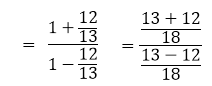= 25/ 1

= 25

6.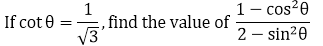Solution:

Given,

cot θ = 1/√3

Using cosec2 θ − cot2 θ = 1, we can find cosec θ

cosec θ = √(1 + cot2 θ)

= √(1 + (1/√3)2)

= √(1 + (1/3)) = √((3 + 1)/3)

= √(4/3)

⇒ cosec θ = 2/√3

So, sin θ = 1/ cosec θ = 1/ (2/√3)

⇒ sin θ = √3/2

And we know that

cos θ = √(1 – sin2 θ)

= √(1 – (√3/2)2)

= √(1 – (3/4))

= √((4 – 3)/4)

= √(1/4)

⇒ cos θ = 1/2

Now, using cos θ and sin θ in the expression, we have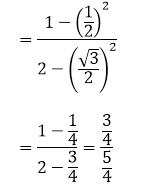= 3/5

7.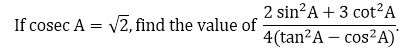Solution:

Given,

cosec A = √2

Using cosec2 A − cot2 A = 1, we find cot A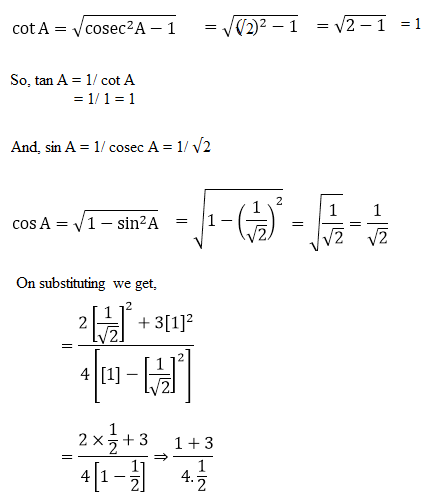= 4/2

= 2

## Frequently Asked Questions on RD Sharma Solutions for Class 10 Maths Chapter 6

Q1

### Why should we follow RD Sharma Solutions for Class 10 Maths Chapter 6 during exam preparation?

RD Sharma Solutions for Class 10 Maths Chapter 6 provides thorough knowledge of concepts to assist students in effective learning. The solutions are designed by our faculty at BYJU’S with the aim of improving academic performance among students. Those who want to clear their doubts quickly are suggested to download the solutions in a PDF format as per their needs. Practising these solutions on a daily basis help students to reduce their stress and boost confidence in solving problems with ease.
Q2

### Why should we download RD Sharma Solutions for Class 10 Maths Chapter 6 from BYJU’S?

BYJU’S provides the most accurate answers for the questions present in the RD Sharma Solutions for Class 10 Maths Chapter 6. Students can download these solutions in PDF format, both in online and offline modes, as per their requirements. The solutions in this chapter are explained by experts very clearly, with neat diagrams wherever necessary. The main objective of developing these solutions is to make learning fun and interesting among students. The accurate solutions help students to clear the confusion that they come across while revising the problems.
Q3

### Are RD Sharma Solutions for Class 10 Maths Chapter 6 important for the exam?

Yes, RD Sharma Solutions for Class 10 Maths Chapter 6 are important for the exam, as it continues in higher grades. High professional teachers recommend students to follow RD Sharma Solutions in a descriptive manner to improve their skills in the respective subject. Diligent practice of these solutions not only clears doubts but also helps to procure high marks in board exams.